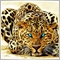# Opening orders 2 or 3 candles after MA cross65

Guys i need help with the following code...

I am trying to open a buy order 2 or 3 bars after fast ma crosses slow ma and when the rsi is greater than 50 as well as when both stochastic lines are greater than 50. A sell order for the opposite. The code below is an attempt at a buy order for the 1 minute period, however, it doesn't open any orders.

```if(Period()==1)
{
{
if(getM1_MA(FastMAPeriod,1)>getM1_MA(SlowMAPeriod,1))
{
if(getM1_MA(FastMAPeriod,2)>getM1_MA(SlowMAPeriod,2))
{
if((getM1_MA(FastMAPeriod,1)-getM1_MA(SlowMAPeriod,1))>MathAbs(UsePoint))
{
if(M1_FastStoc>50)
{
if(M1_SlowStoc>50)
{
if(M1_RSI>50)
{
if(getM1_MA(FastMAPeriod,3)<getM1_MA(SlowMAPeriod,3)
|| (getM1_MA(FastMAPeriod,4)<getM1_MA(SlowMAPeriod,4)))
{
if(OrderSelect(M1_SellTicket,SELECT_BY_TICKET))
{
if(OrderCloseTime()==0)
{
if(M1_SellTicket>0)
{
double M1_CloseLots=OrderLots();
bool M1_Closed=OrderClose(M1_SellTicket,M1_CloseLots,
M1_ClosePrice,UseSlippage,Red);
if(M1_Closed)
{
Alert(Symbol()," M1 SELL Closed");
}
}
}
}
// Calculate stop loss and take profit
if(StopLoss>0) double M1_BuyStopLoss=M1_OpenPrice -(StopLoss*UsePoint);
// Open buy order
M1_SellTicket=0;
}
}
}
}
}
}
}
}

double getM1_MA(int bar_period,int bar_index)
{
return iMA(Symbol(),PERIOD_M1,bar_period,0,MODE_EMA,PRICE_CLOSE,bar_index);
}```